×#### Thank you for registering.

One of our academic counsellors will contact you within 1 working day.

Click to Chat

1800-1023-196

+91-120-4616500

CART 0

• 0

MY CART (5)

Use Coupon: CART20 and get 20% off on all online Study Material

ITEM
DETAILS
MRP
DISCOUNT
FINAL PRICE
Total Price: Rs.

There are no items in this cart.
Continue Shopping```Probability

Table of Content

Introduction to Probability

Mutually Exclusive

Not Mutually Exclusive

Conditional Probability

Some Basic Rules

Complement of an Event

Intersection and Union of Sets of Events

For Second Lottery

Introduction to ProbabilityProbability is an important topic in the mathematics syllabus of the IIT JEE. The word probability refers to the measure of possibility of occurrence of an event. The value of probability can range between 0 and 1. Higher degree of probability implies that the event is most likely to happen.

If n represents the total number of equally mutually exclusive and exhaustive possible outcomes of an experiment and m of them are favorable to the event A, then the probability of the event A is defined as

P (A) = n (E)/ n(S) = m/n

This is known as classical definition of probability

Mutually Exclusive

If either event A or event B or both events occur on a single performance of an experiment this is called the union of the events A and B denoted as P(A ∪ B). Two events are said to be mutually exclusive if the occurrence of one event does not affect the occurrence of the other.

Therefore, if there are n possible outcomes of an experiment which are mutually exclusive, exhaustive and equally likely then, since the probability associated with each outcome is the same (say x) and since they are mutually exclusive, the probability of occurrence of one of them is the sum nx which must be equal to 1(because they are also exhaustive).

That is, nx = 1 => x = 1/n.

If two events are mutually exclusive then the probability of either occurring is

P (A or B) = P(A ∪ B) = P (A) + P (B).

If E1, E2,….., En are mutually exclusive events, then

P(E1 ∪ E2 ∪  ...... UEn) =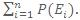For example, the chance of rolling a 3 or 5 on a die is

P (3 or 5) = P (3) + P (5) = 1/6 +1/6 = 1/3

Not Mutually Exclusive

If the occurrence of one event affects or is dependent on the occurrence of the other then the events are not mutually exclusive.

If the events are not mutually exclusive then

P (A or B) = P (A) +P (B) – P (A and B)

For example, when drawing a single card at random from a regular deck of cards, the chance of getting a heart or a face card (J,Q,K) (or one that is both) is given by 13/52 + 12/52 – 3/52 = 11/26, because of the 52 cards of a deck 13 are hearts, 12 are face cards, and 3 are both: here the possibilities included in the "3 that are both" are included in each of the "13 hearts" and the "12 face cards" but should only be counted once.

Conditional Probability

The probability of occurrence of some event A, given that the event B has already occurred is called the conditional probability. Conditional probability is written as P (A| B), and is read as "the probability of A, given B". It is defined by

P (A | B) = P (A∩ B) / P (B)

As is clear from the above expression that if P (B) = 0, then P (A| B) is undefined.

For instance, consider a bag containing 4 rings, 2 orange and 2 purple. The probability of taking out an orange ring is ½, but when the second ring is selected, the probability of it being either an orange ring or a purple ring depends on the ring previously taken, such as, if an orange ring was taken, the probability of picking a orange ring again would be 1/3 since only 1orange and 2purple rings would be remaining.

Some Basic Rules

(1) P (Φ) = 0, P (Ω) = 1.

Φ and Ω are both events and hence their probabilities are defined. The event Φ denotes the event that is impossible and never happens and hence its probability is zero. The event Ω has probability 1 and is certain or necessary. In general, P (A) is the probability of event A; "A takes place or occurs with probability P (A)."

(2) The probability function (or, as it is most commonly called, measure) is required to be additive: for two disjoint events A and B, i.e. whenever A ∩ B = Φ, P (A∪B) = P (A) + P (B)

(3) P (A∪B) = P (A) + P (B) - P (A ∩ B).

This result can also be derived from the previous result. In fact, by assuming all the sets A-B, B-A, A∩ B are disjoint, we get:

P(A) +P(B) = [P(A-B) + P(A∩B) ] + [P(B-A) + P(A∩B)]

= P (A-B) + P (A∩B)] + [P (B-A) + P (A∩B)]

= P (A∪B) + P (A ∩ B)

which is exactly the required result.

(4) P (∪i ≥1Ai) = ∑i ≥1P (Ai).

Complement of an Event

The complement of an event A is the set of all outcomes that is not in A. The complement of event A is expressed as Ac.

The probability of an event and its complement always add up to 1 (An event either occurs or it doesn't occur).

P (A) + P (Ac) = 1

P (Ac) = 1 − P (A)

Some key points:

(i)     For any event A, A⊆S.

(ii)    0 < P(A) < 1, P(A) ∈ R

(iii)    P (A) + P (Ac) = 1

(iv)   If ‘a’ cases are favorable to an event A and ‘b’ cases are favorable to an event Ac (i.e. unfavorable to A) then P (A) = a/ (a+b) and P (Ac) = b/ (b+a).

We say that odds in favor of A are a: b

And odds against of A are b: a

(v)  Since A and A' are mutually exclusive events, P (AUA') = P (A') =1.

Notation:  Let A and B by two events, then

(i)     A' oror Ac stands for the non-occurrence of negation of A.

(ii)    AUB stands for the occurrence of at least one of A or B.

(iii)    A∩B stands for the simultaneous occurrence of A and B.

(iv)   A'∩ B' stands for the non-occurrence of both A and B.

(v)    A ⊆ B stands for "the occurrence of A implies occurrence of B".

Illustration:

A die is rolled. What is the probability that outcome will be an even number?

Solution:

When the die is rolled the possible outcomes are

S = {1, 2, 3, 4, 5, 6,} and A = outcome will be even number i.e.
{2, 4, 6}

So, P (A) = n (E)/n(S) = 3/6 = 1/2

Illustration:

Two dice are thrown simultaneously. What is the probability of obtaining a total score of seven?

Solution:

There are six possible ways as to the number of points on the first die; and to each of these ways, there corresponding 6 possible numbers of points on second die. Hence total number of ways

S = 6 × 6 = 36

We now find out how many ways are favorable to the total of 7 points. This may happen only in following ways: (1, 6), (6, 1), (2, 5), (5, 2), (3, 4), (4, 3).

Hence, required Probability = 6/36 =1/6.

Illustration:

Suppose a die is rolled, can we find the probability that either odd number or a number divisible by 4 comes?

Solution:

When a die is rolled 1, 2, 3, 4, 5 and 6 are possible outcomes. So,
S = {1, 2, 3, 4, 5, 6}.

Let   event A: odd number i.e. A = {1, 3, 5}

event B: number divisible by 4 i.e. B = {4}

Here, we have represented the sample space and event in Venn diagram.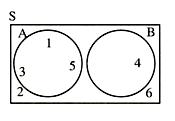We have to find probability of either A or B, i.e. P(A U B)

Clearly, A U B = {1, 3, 4, 5} i.e. A + B

Hence, P (A U B) = P (A) + P (B)

= 3/6 +1/6 = 2/3.

Now, think about a different situation: A die is rolled, can we calculate the probability that the number, which comes is either odd or divisible by 3?

Sample space is S = {1, 2, 3, 4, 5, 6}.

Define, event A: odd number i.e. A = {1, 3, 5}

event B : divisible by 3 i.e. B = {3, 6}

We can represent sample space and even in Venn diagram.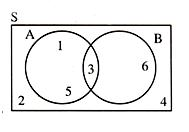Now, we have to find probability that either A or B occurs i.e. P(A U B)

Clearly, A U B = P{1, 3, 5, 6}

Can we write A U B = A + B?

Let's do it, A U B = 1, 3, 5, 3, 6; this is incorrect for obvious reasons since we counted 3 twice.

From above illustration, it can be concluded that when we write
A U B = A + B we count the common element twice, once while counting the elements of A and once while counting the elements of B. Therefore in order to get correct result we should subtract it (common) element once i.e.

A U B = A + B - A B {A  B element common in both A and B}

= 1, 3, 5, 4, 6, - 3

= 1, 3, 5, 6

Hence the probability

P(A U B) = P(A) + P(B) - P(A  B) = (3/6) + (2/6) - (1/6) = 2/3

Note:

1. In previous case, P (AUB) = P (A) + P (B) was incorrect because A∩B ≠ ∅. So, we get a very important result.

P (AUB) = P (A) + P (B) when A and B are mutually exclusive events.

P (AUB) = P (A) + P (B) - P (A∩B), when A and B are not mutually exclusive events.

2. This particular case of two events can easily be generalized for the three events A, B and C

P (AUBUC) = P(A) + P(B) + P(C) - P(A∩B) - P(B∩C) - P(C∩A) + P(A∩B∩C).

Illustration:

The probabilities that a student passes in Mathematics, Physics and Chemistry are m, p and c respectively. Out of these subjects, the student has a 75% chance of passing in at least one, a 50% chance of passing in at least two and a 40% chance of passing in exactly two. Prove that m + p + c = 27/20.

Solution:

From the given information we derive the following equations

1 - (1 - m) (1 - p) (1 - c) = 0.75                               ............ (i)

(1 - m) pc + m (1 - p) c + mp (1 - c) + mpc = 0.5              ............ (ii)

(1 - m) pc + m (1 - p) c + mp (1 - c) = 0.4                  ........... (iii)

(ii) - (iii) gives : mpc = 0.1 = 1/10 From equation (i), we get

p + c + m - (mp +mc + pc) + mpc = 0.75                    ............. (iv)

From (ii),

pc + mc + mp - 3mpc + mpc = 0.5

=> pc + mc + mp = 0.7

Substituting in (iv), we get

m + p + c = 0.75 - 0.1 + 0.7 = 1.35 = 135/100 = 27/20

Intersection and Union of Sets of Events

Let E1, E2, ......, En be n events, then P(E1UE2U ...... UEn), represents the probability of occurrence of at least one of the events from E1, E2, ......, En and P(E1∩E2∩ ...... ∩En) represents the occurrence of all the events together.

In general, we have

P(E1UE2U ... UEn)=P(Ei∩Ej∩Ek)-...+(-1)n-1P(E1∩E2∩...∩En).

Corollary: If A and B are any two events,

P (AUB) = P (A) + P (B) - P (A∩B).

Note that P (A∩B') = P (A) - P (A∩B).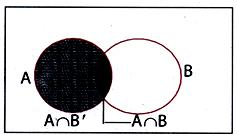Also for any two events A and B,

P(exactly one of them occurs)

= P(E1) + P(E2) - P(E1∩E2)

= P(E1UE2) - P(E1∩E2).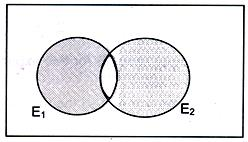If E1, E2 and E3 be three events, then

(i)  P (at least two of E1, E2, E3 occur)

= P (E2∩E3) + P (E3∩E1) + P (E1∩E2) - 2P (E1∩E2∩E3),

(ii) P (exactly two of E1, E2, E3 occur)

= P (E2∩E3) + P (E3∩E1) + P(E1∩E2) - 3P(E1∩E2∩E3),

(ii) P (exactly one of E1, E2, E3 occur)

= P(E1) + P(E2) + P(E3) - 2P(E3∩E1) + 2P(E1∩E2) + 3P(E1∩E3).

If events are mutually exclusive, P (E1UE2U ...... UEn) =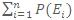.

Illustration:

There are 4red, 5 green and 1 blue marble and 2 black marbles in the bag. Suppose you select one marble at random. Find each probability:

P (Black), P (Red), P (Red or Black), P (not blue), P (not purple).

Solution:

There are 12 marbles in total and so sample space =12. We know by the definition that

Probability= Number of ways a certain outcome can occur/ Total possible outcomes (Sample Space)

Now there are four red balls in the bag. So P (Red) = 4/12=1/3

Similarly, there are 2 black balls so P (Black) = 2/12 = 1/6.

P (Blue) = 1/12.

So P (not Blue) = 1-1/12 = 11/12.

P (Red or Black) = P (Red) + P (Black) = 4/12+2/12 = 6/12 =1/2.

P (not Purple) = 1.

Since there is no purple marble in the bag so there are 100% chances of selecting a non-purple marble.

Illustration:

In a single cast with fair dice, what is the chance of throwing

(i)     two 4's,                      (ii)    a doublet,

(iii)    five - six,                   (iv)   a sum of 7.

Solution:

(i)     There are 6 × 6 equally likely cases

(as any face of any die may turn up)

=>     36 possible outcomes. For this event, only one outcome (4, 4) is favourable

=>     probability = 1/36

(ii)    A doublet can occur in six ways {(1, 1), (2, 2), (3, 3), (4, 4),
(5, 5), (6, 6)}

Therefore probability of a doublet = 6/36 = 1/6.

(iii)   Two favourable outcomes {(5, 6), (6, 5)}:

This probability = 2/36 = 1/18.

(iv)    A sum of 7 can occur in the following cases: {(1, 6), (2, 5),
(3, 4), (4, 3), (5, 2), (6, 1)}which are 6 in number. Therefore probability = 6/36 = 1/6.

Illustration:

Tickets numbered 1 to 20 are mixed up and then a ticket is drawn at random. What is the probability that the ticket drawn has a number which is a multiple of 3 or 5?

Solution:

Here, S = {1, 2, 3, 4, ...., 19, 20}.

Let E = event of getting a multiple of 3 or 5 = {3, 6, 9, 12, 15, 18, 5, 10, 20}.

Henec, P (E) = n (E) / n(S) = 9/20.

Illustration:

Seven accidents occur in a week. What is the probability that they happen on the same day.

Solution:

Total number of cases = Total no. of ways in which 7 accidents can happen in a week = 77.

Favourable number of cases out of these = number of those in which all 7 happen on one day (any day of the week) = 7. Hence the required probability = 7/77 = 1/76.

Illustration:

From a bag containing 5 white, 7 red and 4 black balls a man draws 3 balls at random. Find the probability that all are white.

Solution:

Total number of balls in the bag = 5 + 7 + 4 = 16

=> The total number of ways in which 3 balls can be drawn

= 16C3 = (16*15*14)/ (3*2*1) = 560.

Thus sample space S for this experiment has 560 outcomes

i.e. n(S) = 560.

Let E be the event of all the three balls being white. Total number of white balls in the bag is 5.

So the number of ways in which 3 white balls can be drawn = 5C3
= (5*4*3)/ (3*2*1) = 10.

Thus E has 10 elements of S, i.e. n(E) = 10

=> Probability of E, P(E) = n(E)/n(S) = 10/560 = 1/56.

Illustration:

From a pack of 52 cards two cards are drawn at random. Find the probability of the following events:

(i)     Both the cards are spades.

(ii)    One card is of spade and the other is a diamond.

Solution:

The total number of ways in which 2 cards can be drawn

= 52C2 = (52*51)/(1*2) = 26 × 51 = 1326.

=> Number of elements in the sample space S are n(S) = 1326.

(i)     Let the event that both cards are of spade, be denoted by E1. Then n(E1) =  Number of elements in E1 = Number of ways in which 2 cards  can be selected out of 13 cards of spade = 13C2 = (13*12)/(1*2) = 78.

Hence the probability of E1 = P(E1) = n(E1)/n(S) = 78/1326 = 1/17.

(ii)    Let E2 be the event that one card is of spade and one is of diamond. Then n(E2) =  number of elements in E2 = number of ways in which one card of spade can be selected out of 13 spades and one card of diamond can be selected out of 13 diamond cards

= 13C1 × 13C1 = 13×13 = 169

=> P(E2) = n(E2)/n(S) = 169/1326 = 13/102.

Illustration:

A has 3 shares in a lottery containing 3 prizes and 6 blanks: B has one share in a lottery containing one prize and 2 blanks. Compare their chances of success.

Solution:

Total number of tickets in the first lottery = 3 + 6 = 9

A may select any three tickets out of these 9 tickets. Therefore, the number of element in the sample space S is given by

= n(S) = 9C3 = (9*8*7)/(3*2*1) = 84.

Let E1 be the event of winning the prize in the first lottery by A. So,.E1c is the event of not winning the prize by A. Then number of elements

in E1c is given n(E1c) = number of ways of selecting 3 tickets out of six blank tickets = 6C3 = (6*5*4)/(1*2*3) = 20.

=> The probability of not winning the prize by A = P(E1c ) = n(E1c )/n(S) = 20/84 = 5/21.

Since P (E1) + P (E1c ) = 1, P(E1) = 1 - P(E1c ) or P(E1) = 1 - 5/21 = 16/21.

For Second Lottery

n(S) = number of ways of selecting 1 ticket out of 3 tickets.

Or n(S) = 3C1 = 3.

Let E2 be the event of winning the prize by B.

n(E2) = number of ways of selecting one ticket out of 1 prize ticket
= 1C1 = 1

=> The probability of winning the prize by B

= P(E2) = n(E2)/n(S) = 1/3 so that the ratio of the probabilities of winning the prizes by A and B = P(E2)/P(E2) = (16/21)/(1/3) = 16/7.

Illustration:

From a pack of cards, four are drawn at random. What is the chance that there is one card of each suit?

Solution:

A pack of cards has 52 cards. Let S be the sample space. Then

n(S) = 52C4 = (52*51*50*49)/(4*3*2*1) = 13 × 17 × 25 × 49.

Let E be the event of drawing one card from each suit.

There are four different suits and each suit has 13 cards.

n(E) = number of total ways of drawing one card from each suit.

= 13C1 × 13C1 × 13C1 × 13C1 = 13 × 13 × 13 × 13

ð Required probability = P(E) = n(E)/n(S) =(13*13*13*13)/(13*17*25*49)

= 2197/20825.

View the video for more examples on probability theory

Illustration:

In a given race the odds in favour of four horses A, B, C, D are 1:3, 1:4, 1:5, 1:6 respectively. Assuming that, a dead heat is impossible, find the chance that one of them wins the race.

Solution:

Let P(A), P(B), P(C) and P(D) be the responsibilities of winning of the horses A, B, C and D respectively. Then

P(A) = 1/4, P(B) = 1/5, P(C) = 1/6, P(D) = 1/7.

Since the above events are mutually exclusive, the chance that one of them wins

= P(AUBUCUD) = P(A) + P(B) + P(C) + P(D)

= (1/4) + (1/5) + (1/6) + (1/7).

Illustration:

Ten passengers get into an elevator on the ground floor of a 20 floor building. What is the probability that they will all get out at different floors?

Solution:

Total no. of ways in which the 10 passengers can get out = 2010.

Favourable no. of ways = number of those cases in which not more than one passenger gets out at each floor = 20P10 = 20! /10!

Required probability = 20!/(10!(20)10).

You may consult the Sample Papers to get an idea about the types of questions asked.

Illustration:

There are three events A, B and C out of which one and only one can happen. The odds are 8 to 3 against A, 5 to 2 against B. Find odds against C.

Solution:

Let the total no. of cases = m + n + p:

m are in favour of A, n in favour of B & p in favour of C.

We have (n+p)/m = 8/3, (m+p)/n = 5/2, so that

P (A') = (n+p)/(m+n+p) = 8/11,  P(B') = (m+p)/(m+n+p) = 5/7

=> P (A) = 3/11, P (B) = 2/7

Also P(A) + P(B) + P(C) = 1 => P(C) = 34/77

To read more, Buy study materials of Probability comprising study notes, revision notes, video lectures, previous year solved questions etc. Also browse for more study materials on Mathematics here.
```### Course Features

• 731 Video Lectures
• Revision Notes
• Previous Year Papers
• Mind Map
• Study Planner
• NCERT Solutions
• Discussion Forum
• Test paper with Video Solution